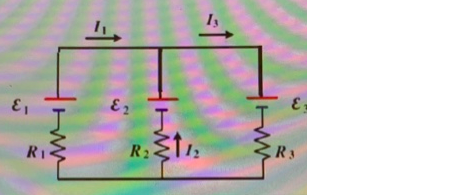# Problem: For the circuit shown in Fig. let R1 = 5 Ω, R2 = 15 Ω, R3 = 10 Ω, ε1  = 60 V, ε2 = 80 V, ε3 = 10 V and I1 = 2A. Find the value of the current I2 in the circuita. 4 Ab. 2 Ac. - 2 Ad. - 1 A

###### FREE Expert Solution

We're going to use Kirchhoff's loop rule, which is:

$\overline{){\mathbf{\Sigma }}{\mathbf{V}}{\mathbf{=}}{\mathbf{0}}}$

We'll also use Ohm's law to help get the voltages.

From Ohm's law:

$\overline{){\mathbf{V}}{\mathbf{=}}{\mathbf{i}}{\mathbf{R}}}$, where i is the current and R resistance.

79% (352 ratings)###### Problem Details

For the circuit shown in Fig. let R1 = 5 Ω, R2 = 15 Ω, R3 = 10 Ω, ε1  = 60 V, ε2 = 80 V, ε3 = 10 V and I1 = 2A. Find the value of the current I2 in the circuita. 4 A

b. 2 A

c. - 2 A

d. - 1 A

Frequently Asked Questions

What scientific concept do you need to know in order to solve this problem?

Our tutors have indicated that to solve this problem you will need to apply the Kirchhoff's Loop Rule concept. You can view video lessons to learn Kirchhoff's Loop Rule. Or if you need more Kirchhoff's Loop Rule practice, you can also practice Kirchhoff's Loop Rule practice problems.